# Electric Dollar Store

•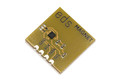•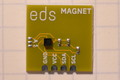•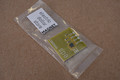•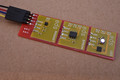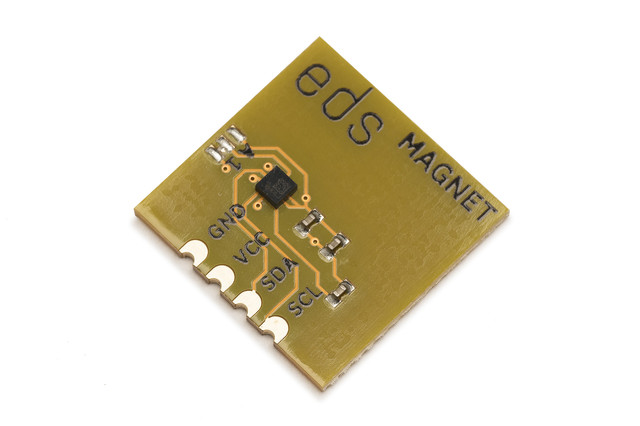• ## I²C 3-Axis Digital Magnetometer

• LIS3MDL digital 3D compass
• selectable scales of ±2, ±4, ±8 or ±16 gauss
• 16-bit data output
• standard 18x18mm module with I²C and power

• \$1.80

The LIS3MDL is an ultra-low-power high-performance three-axis magnetic sensor. In typical use it delivers a 16-bit 3D (X,Y,Z) magnetic field reading 80 times per second.

The sensor is factory calibrated for sensitivity and zero-gauss level. The trimming values are stored in the device in non-volatile memory. Each time the device is turned on, the trimming parameters are downloaded to the calibration registers. So the device can be used without further calibration.

```import sys
import struct
import time

from i2cdriver import I2CDriver, EDS

if __name__ == '__main__':
i2 = I2CDriver(sys.argv)

d = EDS.Magnet(i2)
while 1:
print(d.measurement())
```
```#include <Wire.h>

class magnet {
uint8_t a;
byte status;
public:
int16_t x, y, z;
void begin(byte _a = 0x1c) {
a = _a;
regwr(0x22, 0); // CTRL_REG3 operating mode 0: continuous conversion
}
void regwr(byte addr, byte val) {
Wire.beginTransmission(a);
Wire.write(val);
Wire.endTransmission();
}
int16_t rd16() {
return r;
}
do {
Wire.beginTransmission(a);
Wire.write(0x27);
Wire.endTransmission(false);
Wire.requestFrom(a, (uint8_t)7);
x = rd16();
y = rd16();
z = rd16();
} while ((status & 8) == 0);
}
};

magnet Magnet;

void setup() {
Serial.begin(115200);
Wire.begin();
Magnet.begin();
}

void loop() {
Serial.print(Magnet.x); Serial.print(' ');
Serial.print(Magnet.y); Serial.print(' ');
Serial.print(Magnet.z); Serial.println();
}
```
```from machine import I2C
import struct
import time

class Magnet:
""" MAGNET is an ST LIS3MDL 3-axis magnetometer """
def __init__(self, i2, a = 0x1c):
self.i2 = i2
self.a = a
self.regwr(0x22, 0) # CTRL_REG3 operating mode 0: continuous conversion

def rd(self):
""" Read the measurement STATUS_REG and OUT_X,Y,Z """

def measurement(self):
""" Wait for a new field reading, return the (x,y,z) """
while True:
(status, x, y, z) = self.rd()
if status & 8:
return (x, y, z)

def main():
i2 = I2C(1, freq = 100000)

d = Magnet(i2)

while True:
print(d.measurement())
```

 Default I²C address `0x1c` (`0b0011100`) Current consumption (typ.) 0.04 mA Vcc 1.9 - 3.6 V